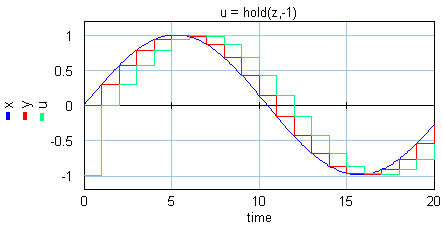﻿ 20-sim webhelp > Language Reference > Functions > Discrete > hold

# hold

## Syntax

y = hold(x,init);

## Description

The hold function implements a zero order hold function operating at a specified sampling rate. It provides a mechanism for creating a continuous output signal y out of a discrete input signal x. The initial value of y is equal to the value of init.## Examples

x = sin(time*0.3);

y = sample(x);

z = previous(y);

u = hold(z,-1);

## Limitations

x and y must be scalars. x be a discrete signal. y is a continuous signal. 20-sim will automatically detect the existence of discrete signals. Each chain of discrete signals will be assigned a specific sampletime. You can set this sample time to any desired value in the Simulator (choose Properties, Simulation and Discrete System).